# Dimension Reduction Methods (1)

## Introduction

In this chapter, we move fully into multivariate analysis and begin by addressing some approaches to reduce the dimensionality of the variable space. These are methods that help to address the so-called curse of dimensionality, a concept originally formulated by Bellman (1961). In a nutshell, the curse of dimensionality means that techniques that work well in small dimensions (e.g., k-nearest neighbor searches), either break down or become unmanageably complex (i.e., computationally impractical) in higher dimensions.

After a brief example to illustrate the curse of dimensionality in concrete terms, we move on to principal components analysis (PCA), a core method of both multivariate statistics and machine learning. Dimension reduction is particularly relevant in situations where many variables are available that are highly intercorrelated. In essence, the original variables are replaced by a smaller number of proxies that represent them well.

In addition to a standard description of PCA and its implementation in GeoDa, we also introduce a spatialized approach, where we exploit geovisualization, linking and brushing to illustrate how the dimension reduction is represented in geographic space.

To illustrate these techniques, we will again use the Guerry data set on moral statistics in 1830 France, which comes pre-installed with GeoDa. Before proceeding with a discussion of the PCA functionality, we briefly illustrate some aspects of the curse of dimensionality.

### Objectives

• Illustrate the curse of dimensionality

• Illustrate the mathematics behind principal component analysis

• Compute principal components for a set of variables

• Interpret the characteristics of a principal component analysis

• Spatialize the principal components

#### GeoDa functions covered

• Clusters > PCA
• select variables
• PCA parameters
• PCA summary statistics
• saving PCA results

## The Curse of Dimensionality

The curse of dimensionality was originally described by Bellman (1961) in the context of optimization problems. Consider the following simple example where we look for the maximum of a function in one variable dimension, as in Figure 1.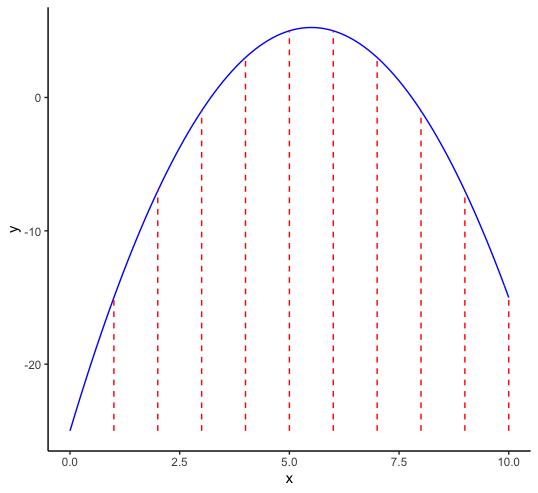Figure 1: Discrete optimization in one variable dimension

One simple way to try to find the maximum is to evaluate the function at a number of discrete values for $$x$$ and find the point(s) where the function value is highest. For example, in Figure 1, we have 10 evaluation points, equally dividing the x-range of $$0-10$$. In our example, the maximum is between 5 and 6. In a naive one-dimensional numerical optimization routine, we could then divide the interval between 5 and 6 into ten more subintervals and proceed. However, let’s consider the problem from a different perspective. What if the optimization was with respect to two variables? Now, we are in a two-dimensional attribute domain and using 10 discrete points for each variable actually results in 100 evaluation points, as shown in Figure 2.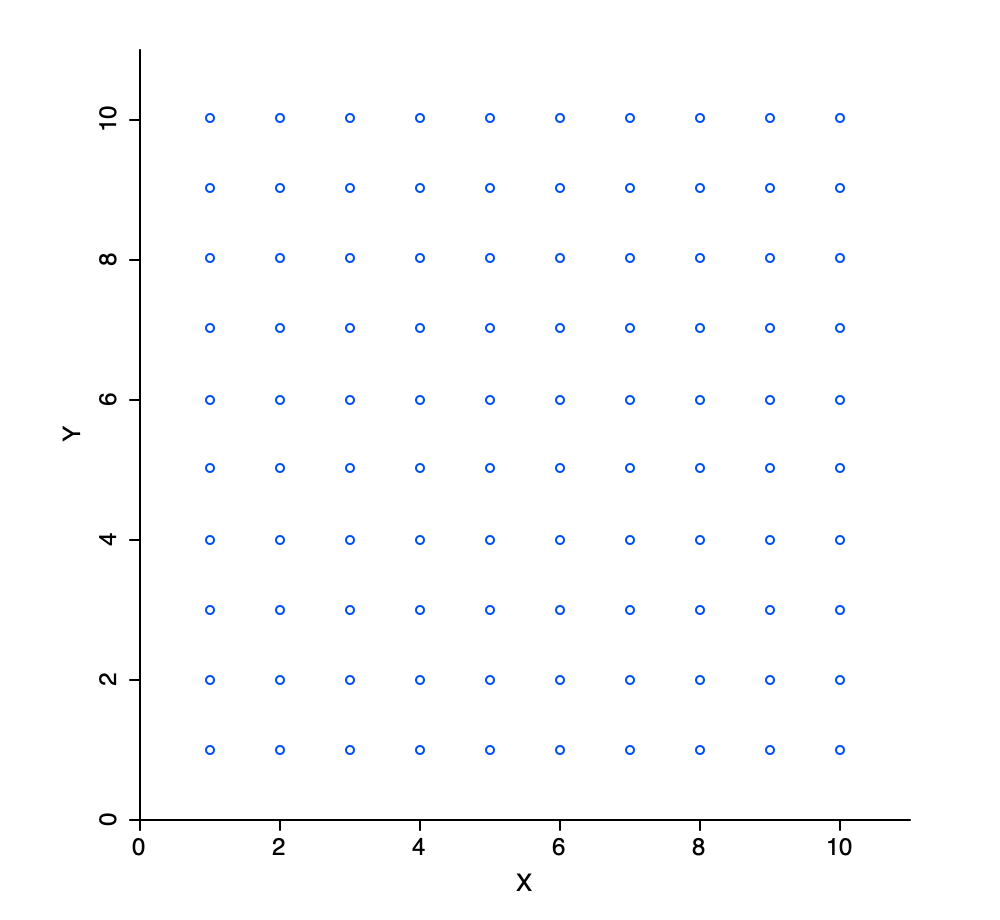Figure 2: Discrete evaluation points in two variable dimensions

Similarly, for three dimensions, there would be $$10^3$$ or 1,000 evaluation points, as in Figure 3.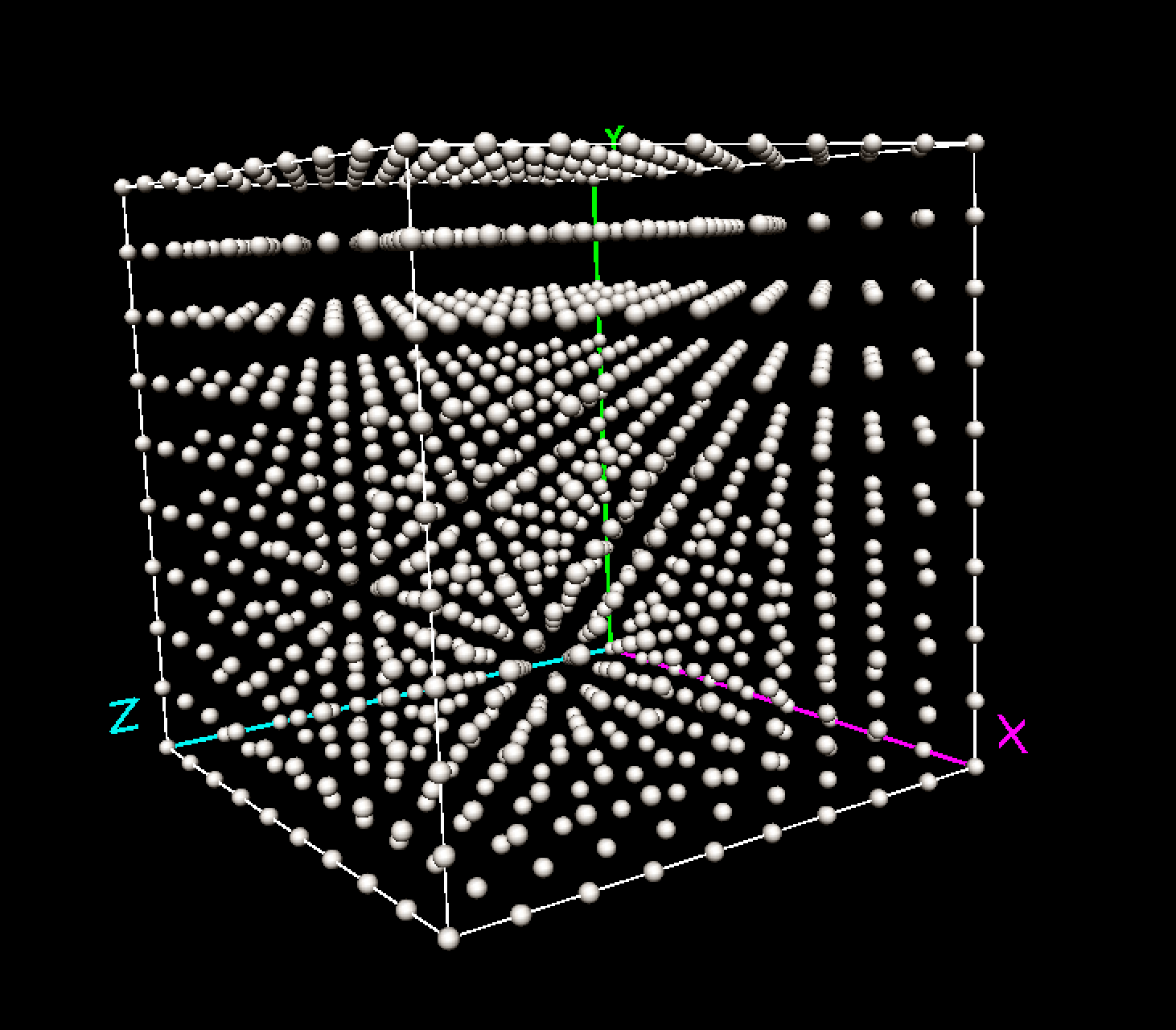Figure 3: Discrete evaluation points in three variable dimensions

In general, if we keep the principle of 10 evaluation points per dimension, the required number of evaluation points is $$10^k$$, with $$k$$ as the number of variables. This very quickly becomes unwieldy, e.g., for 10 dimensions, there would need to be $$10^{10}$$, or 10 billion evaluation points, and for 20 dimensions $$10^{20}$$ or 100,000,000,000,000,000,000, hence the curse of dimensionality. Some further illustrations of methods that break down or become impractical in high dimensional spaces are given in Chapter 2 of Hastie, Tibshirani, and Friedman (2009), among others.

### The Empty Space Phenomenon

A related and strange property of high-dimensional analysis is the so-called empty space phenomenon. In essence, the same number of data points are much further apart in higher dimensional spaces than in lower dimensional ones. This means that we need (very) large data sets to do effective analysis in high dimensions.

To illustrate this point, consider three variables, say $$x$$, $$y$$, and $$z$$, each generated as 10 uniform random variates in the unit interval (0-1). In one dimension, the observations on these variables are fairly close together. For example, in Figure 4, the one-dimensional spread for each of the variables is shown on the horizontal axes of a parallel coordinate plot (PCP). Clearly, the points on each of the lines that correspond to the dimensions are spaced fairly closely together.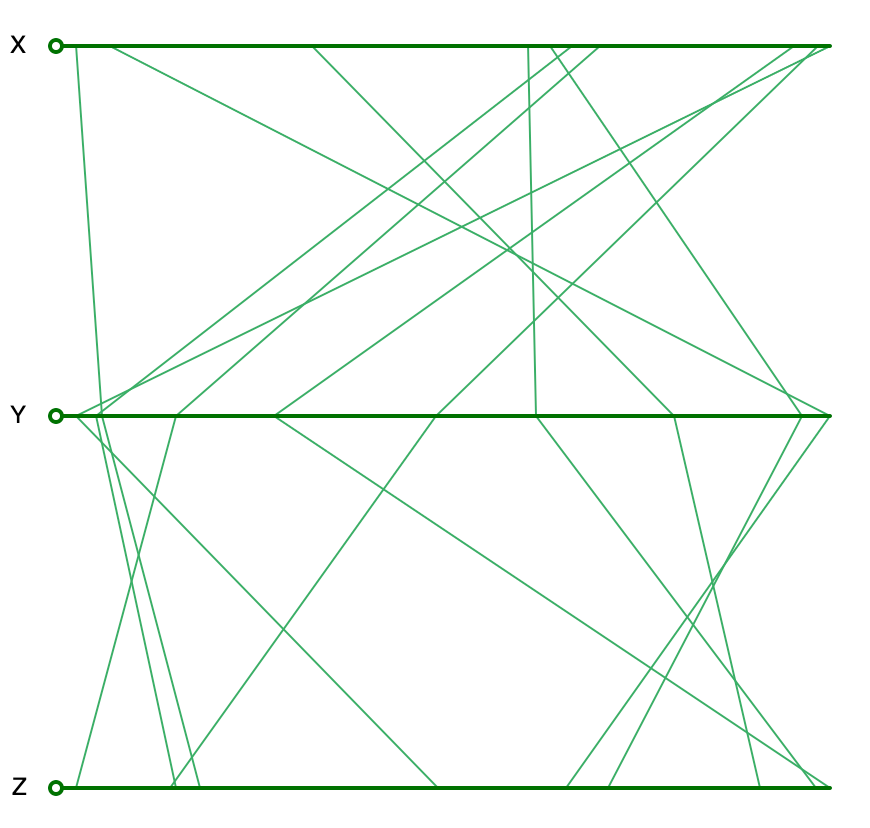Figure 4: Uniform random variables in one dimension

However, when represented in a two-dimensional scatter plot, we observe large empty spaces in the unit quadrant, as in Figure 5 for $$x$$ and $$y$$ (similar graphs are obtained for the other pairs). The sparsity of the attribute space gets even more pronounced in three dimensions, as in Figure 6.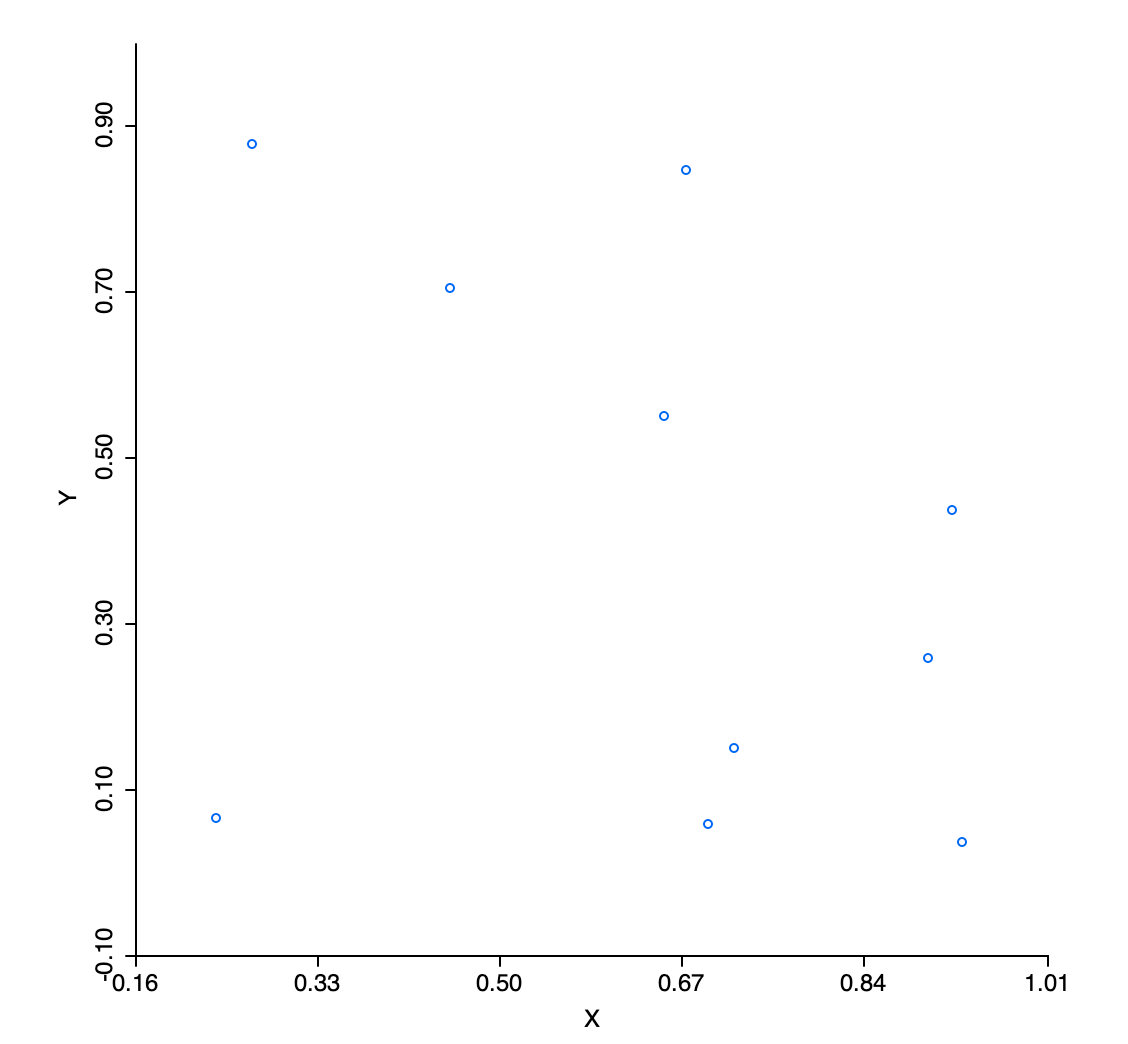Figure 5: Uniform random variables in two dimensions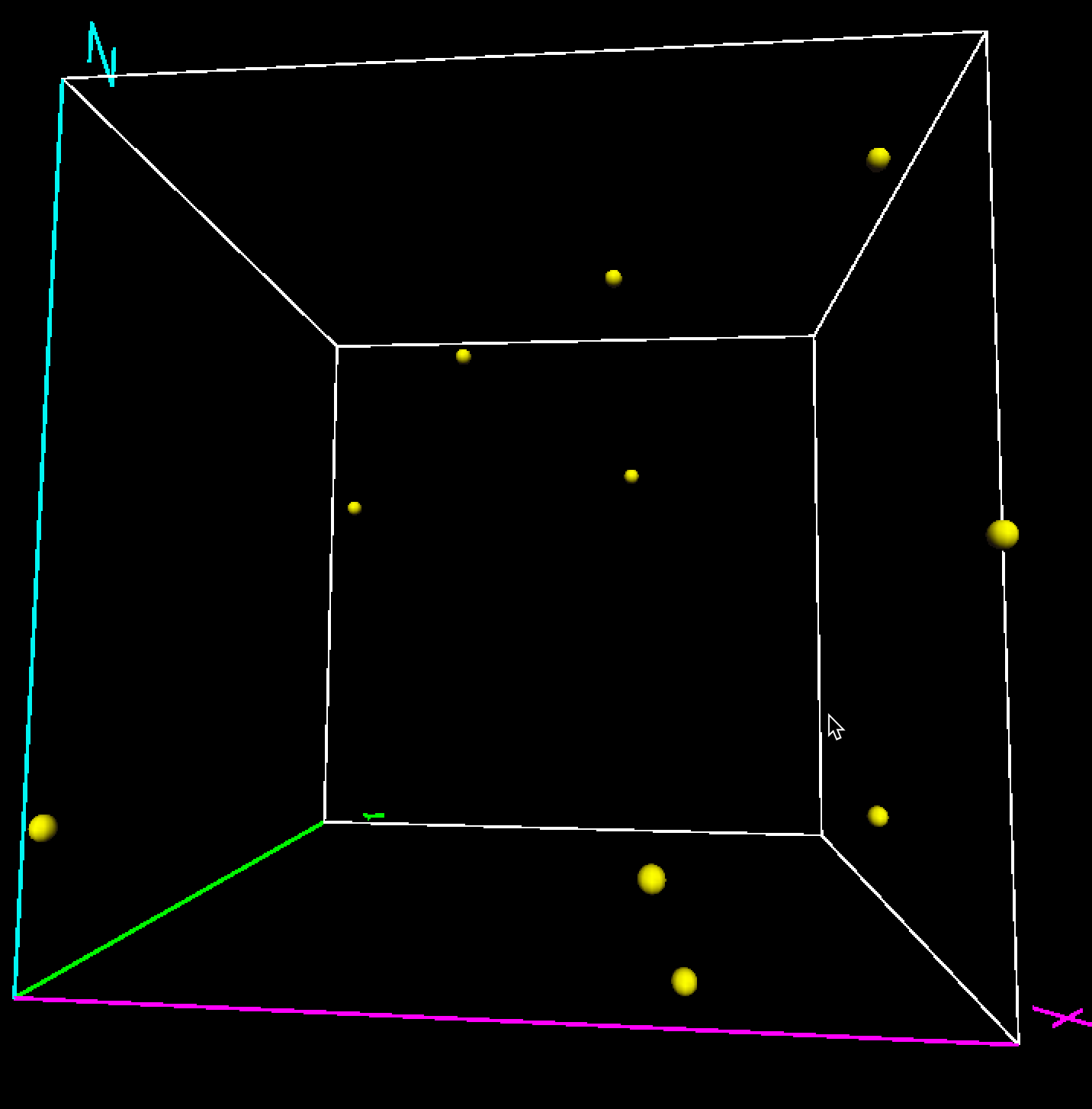Figure 6: Uniform random variables in three dimensions

To quantify this somewhat, we can compute the nearest neighbor distances between the observation points in each of the dimensions.2 In our example (the results will depend on the actual random numbers generated), the smallest nearest neighbor distance in the $$x$$ dimension is between observations 5 and 8 at 0.009. In two dimensions, the smallest distance is between observations 1 and 4 at 0.094, and in three dimensions, it is also between 1 and 4, but now at 0.146. All distances are in the same units, since we are working with a unit interval for each variable.

Two observations follow from this small experiment. One is that the nearest neighbors in a lower dimension are not necessarily also nearest neighbors in higher dimensions. The other is that the nearest neighbor distance increases with the dimensionality. In other words, more of the attribute space needs to be searched before neighbors are found (this is in general a problem with k-nearest neighbor searches in high dimensions).

Further examples of some strange aspects of data in higher dimensional spaces can be found in Chapter 1 of Lee and Verleysen (2007).

## Principal Component Analysis (PCA)

### Principles

Principal components analysis has an eminent historical pedigree, going back to pioneering work in the early twentieth century by the statistician Karl Pearson (Pearson 1901) and the economist Harold Hotelling (Hotelling 1933). The technique is also known as the Karhunen-Loève transform in probability theory and as empirical orthogonal functions or EOF in meteorology (see, for example, in applications of space-time statistics in Cressie and Wikle 2011; Wikle, Zammit-Mangion, and Cressie 2019).

The derivation of principal components can be approached from a number of different perspectives, all leading to the same solution. Perhaps the most common treatment considers the components as the solution of a problem of finding new variables that are constructed as a linear combination of the original variables, such that they maximize the explained variance. In a sense, the principal components can be interpreted as the best linear approximation to the multivariate point cloud of the data.

More formally, consider $$n$$ observations on $$k$$ variables $$x_h$$, with $$h = 1, \dots, k$$, organized as a $$n \times k$$ matrix $$X$$ (each variable is a column in the matrix). In practice, each of the variables is typically standardized, such that its mean is zero and variance equals one. This avoids problems with (large) scale differences between the variables (i.e., some are very small numbers and others very large). For such standardized variables, the $$k \times k$$ cross product matrix $$X'X$$ corresponds to the correlation matrix (without standardization, this would be the variance-covariance matrix).3

The goal is to find a small number of new variables, the so-called principal components, that explain the bulk of the variance (or, in practice, the correlation) in the original variables. If this can be accomplished with a much smaller number of variables than in the original set, the objective of dimension reduction will have been achieved.

We can think of each principal component $$z_u$$ as a linear combination of the original variables $$x_h$$, with $$h$$ going from $$1$$ to $$k$$ such that: $z_u = a_1 x_1 + a_2 x_2 + \dots + a_k x_k$ The mathematical problem is to find the coefficients $$a_h$$ such that the new variables maximize the explained variance of the original variables. In addition, to avoid an indeterminate solution, the coefficients are scaled such that the sum of their squares equals $$1$$.

A full mathematical treatment of the derivation of the optimal solution to this problem is beyond our scope (for details, see, e.g., Lee and Verleysen 2007, Chapter 2). Suffice it to say that the principal components are based on the eigenvalues and eigenvectors of the cross-product matrix $$X'X$$.

The coefficients by which the original variables need to be multiplied to obtain each principal component can be shown to correspond to the elements of the eigenvectors of $$X'X$$, with the associated eigenvalue giving the explained variance. Note that while the matrix $$X$$ is not square, the cross-product matrix $$X'X$$ is of dimension $$k \times k$$, so it is square. See the Appendix for a quick overview of the concept of eigenvalue and eigenvector.

Operationally, the principal component coefficients are obtained by means of a matrix decomposition. One option is to compute the eigenvalue decomposition of the $$k \times k$$ matrix $$X'X$$, i.e., the correlation matrix, as $X'X = VGV',$ where $$V$$ is a $$k \times k$$ matrix with the eigenvectors as columns (the coefficients needed to construct the principal components), and $$G$$ a $$k \times k$$ diagonal matrix of the associated eigenvalues (the explained variance). For further details, see the Appendix.

A second, and computationally preferred way to approach this is as a singular value decomposition (SVD) of the $$n \times k$$ matrix $$X$$, i.e., the matrix of (standardized) observations, as $X = UDV',$ where again $$V$$ (the transpose of the $$k \times k$$ matrix $$V'$$) is the matrix with the eigenvectors of $$X'X$$ as columns, and $$D$$ is a $$k \times k$$ diagonal matrix such that $$D^2$$ is the matrix of eigenvalues of $$X'X$$.4 A brief discussion of singular value decomposition is given in the Appendix.

It turns out that this second approach is the solution to viewing the principal components explicity as a dimension reduction problem, originally considered by Karl Pearson. More formally, consider the observed vector on the $$k$$ variables $$x$$ as a function of a number of unknown latent variables $$z$$, such that there is a linear relationship between them: $x = Az,$ where $$x$$ is a $$k \times 1$$ vector of the observed variables, and $$z$$ is a $$p \times 1$$ vector of the (unobserved) latent variables, ideally with $$p$$ much smaller than $$k$$. The matrix $$A$$ is of dimension $$k \times p$$ and contains the coefficients of the transformation. Again, in order to avoid indeterminate solutions, the coefficients are scaled such that $$A'A = I$$, which ensures that the sum of squares of the coefficients associated with a given component equals one. Instead of maximizing explained variance, the objective is now to find $$A$$ and $$z$$ such that the so-called reconstruction error is minimized.5

Note that different computational approaches to obtain the eigenvalues and eigenvectors (there is no analytical solution) may yield opposite signs for the elements of the eigenvectors (but not for the eigenvalues). This will affect the sign of the resulting component (i.e., positives become negatives). This is the case in GeoDa for the eigen and SVD options, as illustrated below.

In a principal component analysis, we are typically interested in three main results. First, we need the principal component scores as a replacement for the original variables. This is particularly relevant when a small number of components explain a substantial share of the original variance. Second, the relative contribution of each of the original variables to each principal component is of interest. Finally, the variance proportion explained by each component in and of itself is also important.

In addition to these traditional interpretations, we will be particularly interested in spatializing the principal components, i.e., analyzing and visualizing their spatial patterns.

### Implementation

We invoke the principal components functionality from the Clusters toolbar icon, shown in Figure 7.Figure 7: Clusters toolbar icon

From the main menu, we can select Clusters > PCA. The full list of options is shown in Figure 8. The dimension reduction methods are included in the top part (separated from the others by a thin line). PCA is the first item on the list of options.Figure 8: PCA Option

This brings up the PCA Settings dialog, the main interface through which variables are chosen, options selected, and summary results are provided.

#### Variable Settings Panel

We select the variables and set the parameters for the principal components analysis through the options in the left hand panel of the interface. We choose the six same variables as in the multivariate analysis presented in Dray and Jombart (2011): Crm_prs (crimes against persons), Crm_prp (crimes against property), Litercy (literacy), Donatns (donations), Infants (births out of wedlock), and Suicids (suicides) (see also Anselin 2019, for an extensive discussion of the variables). Note that, as in the original Guerry data set, the scale of each variable is such that larger is better. Specifically, the rates are not the usual events/population (like a crime rate, number of crimes over population), but the reverse, population over events. For example, a large value for Crm_prs actually denotes a low crime rate, since it corresponds with more people per crime committed. The chosen variables appear highlighted in the Select Variables panel, Figure 9.Figure 9: PCA Settings panel

The default settings for the Parameters are likely fine in most practical situations. The Method is set to SVD, i.e., singular value decomposition. The alternative Eigen carries out an explicit eigenvalue decomposition of the correlation matrix. In our example, the two approaches yield opposite signs for the loadings of three of the components. We return to this below. For larger data sets, the singular value decomposition approach is faster and is the preferred option.

The Transformation option is set to Standardize (Z) by default, which converts all variables such that their mean is zero and variance one, i.e., it creates a z-value as $z = \frac{(x - \bar{x})}{\sigma(x)},$ with $$\bar{x}$$ as the mean of the original variable $$x$$, and $$\sigma(x)$$ as its standard deviation.

An alternative standardization is Standardize (MAD), which uses the mean absolute deviation (MAD) as the denominator in the standardization. This is preferred in some of the clustering literature, since it diminishes the effect of outliers on the standard deviation (see, for example, the illustration in Kaufman and Rousseeuw 2005, 8–9). The mean absolute deviation for a variable $$x$$ is computed as: $\mbox{mad} = (1/n) \sum_i |x_i - \bar{x}|,$ i.e., the average of the absolute deviations between an observation and the mean for that variable. The estimate for $$\mbox{mad}$$ takes the place of $$\sigma(x)$$ in the denominator of the standardization expression.

Two additional transformations that are based on the range of the observations, i.e., the difference between the maximum and minimum, are the Range Adjust and the Range Standardize options. Range Adjust divides each value by the range of observations: $r_a = \frac{x_i}{x_{max} - x_{min}}.$ While Range Adjust simply rescales observations in function of their range, Range Standardize turns them into a value between zero (for the minimum) and one (for the maximum): $r_s = \frac{x_i - x_{min}}{x_{max} - x_{min}}.$ Whereas these transformations are used less commonly in the context of PCA, they are quite useful in cluster analysis (in GeoDa, all multivariate methods share the same standardization options).

The remaining options are to use the variables in their original scale (Raw), or as deviations from the mean (Demean).

After clicking on the Run button, the summary statistics appear in the right hand panel, as shown in Figure 10. We return for a more detailed interpretation below.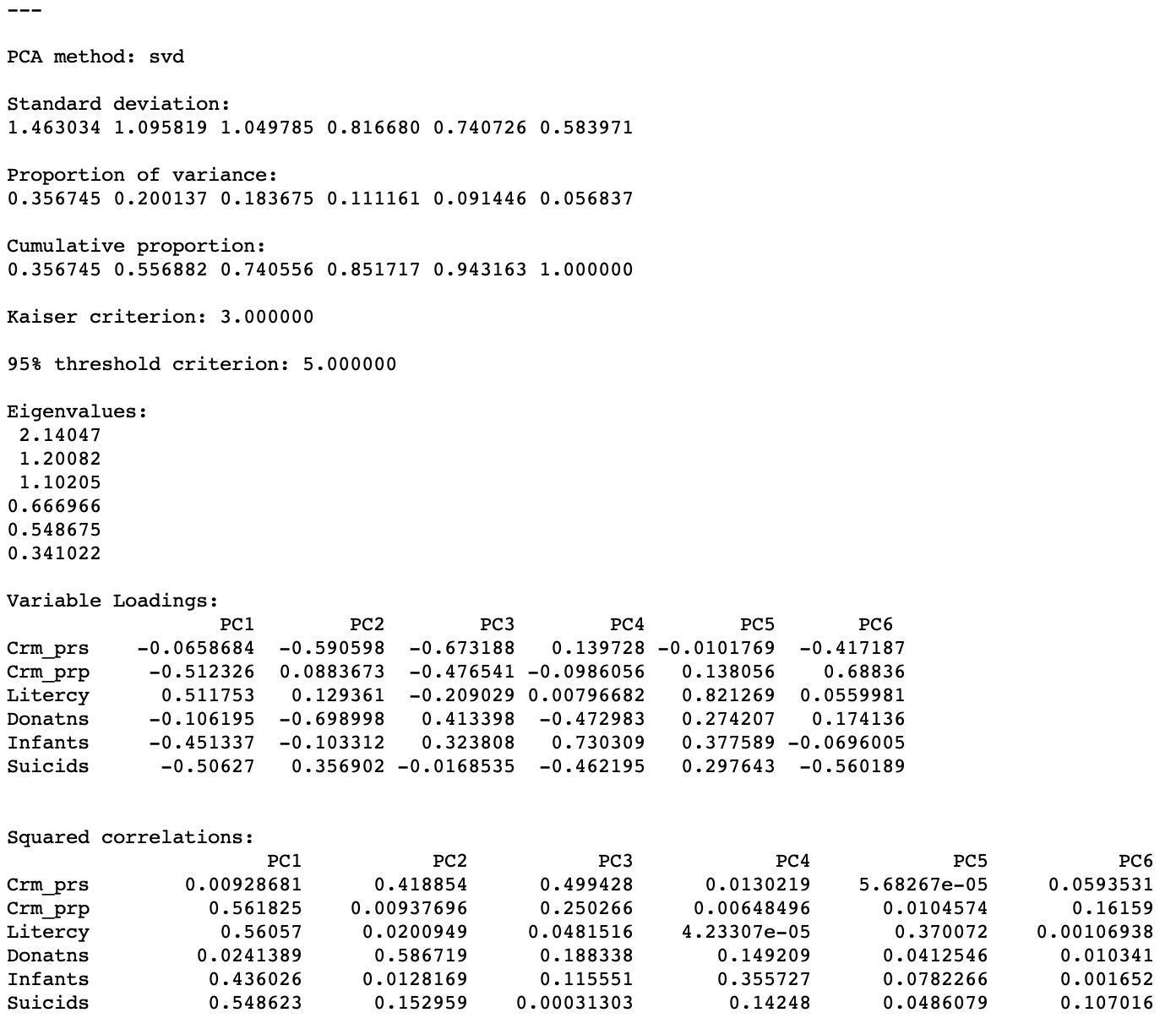Figure 10: PCA results

#### Saving the Results

Once the results have been computed, a value appears next to the Output Components item in the left panel, shown in Figure 11. This value corresponds to the number of components that explain 95% of the variance, as indicated in the results panel. This determines the default number of components that will be added to the data table upon selecting Save. The value can be changed in the drop-down list. For now, we keep the number of components as 5, even though that is not a very good result (given that we started with only six variables).Figure 11: Save output dialog

The Save button brings up a dialog to select variable names for the principal components, shown in Figure 12. The default is generic and not suitable for a situation where a large number of analyses will be carried out. In practice, one would customize the components names to keep the results from different computations distinct.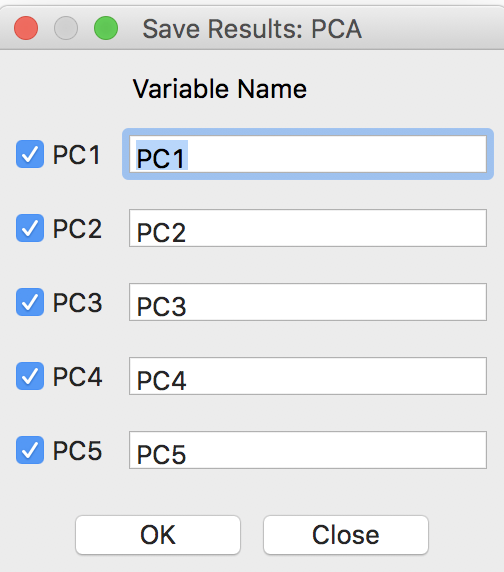Figure 12: Principal Component variable names

Clicking on OK adds the principal components to the data table, as in Figure 13, shown for the first five observations. The principal components now become available for all types of analysis and visualization. As always, the addition is only made permanent after saving the table.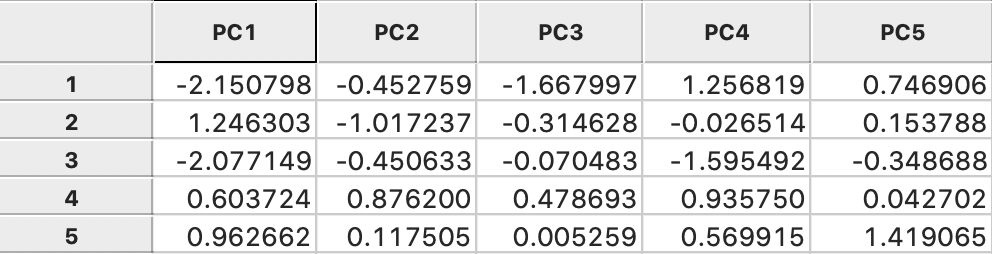Figure 13: Principal Components in the table

### Interpretation

The panel with summary results provides several statistics pertaining to the variance decomposition, the eigenvalues, the variable loadings and the contribution of each of the original variables to the respective components.

#### Explained variance

The first item lists the Standard deviation explained by each of the components. This corresponds to the square root of the Eigenvalues (each eigenvalue equals the variance explained by the corresponding principal component), which are listed as well. In our example, the first eigenvalue is 2.14047, which is thus the variance of the first component. Consequently, the standard deviation is the square root of this value, i.e., 1.463034, given as the first item in the list.

The sum of all the eigenvalues is 6, which equals the number of variables, or, more precisely, the rank of the matrix $$X'X$$. Therefore, the proportion of variance explained by the first component is 2.14047/6 = 0.356745, as reported in the list. Similarly, the proportion explained by the second component is 0.200137, so that the cumulative proportion of the first and second component amounts to 0.356745 + 0.200137 = 0.556882. In other words, the first two components explain a little over half of the total variance.

The fraction of the total variance explained is listed both as a separate Proportion and as a Cumulative proportion. The latter is typically used to choose a cut-off for the number of components. A common convention is to take a threshold of 95%, which would suggest 5 components in our example. Note that this is not a good result, given that we started with 6 variables, so there is not much of a dimension reduction.

An alternative criterion to select the number of components is the so-called Kaiser criterion (Kaiser 1960), which suggests to take the components for which the eigenvalue exceeds 1. In our example, this would yield 3 components (they explain about 74% of the total variance).

The bottom part of the results panel is occupied by two tables that have the original variables as rows and the components as columns.

The first table shows the Variable Loadings. For each component (column), this lists the elements of the corresponding eigenvector. The eigenvectors are standardized such that the sum of the squared coefficients equals one. The elements of the eigenvector are the coefficients by which the (standardized) original variables need to be multiplied to construct each component. We illustrate this below.

It is important to keep in mind that the signs of the loadings may change, depending on the algorithm that is used in their computation, but the absolute value of the coefficients will be the same. In our example, setting Method to Eigen yields the loadings shown in Figure 14.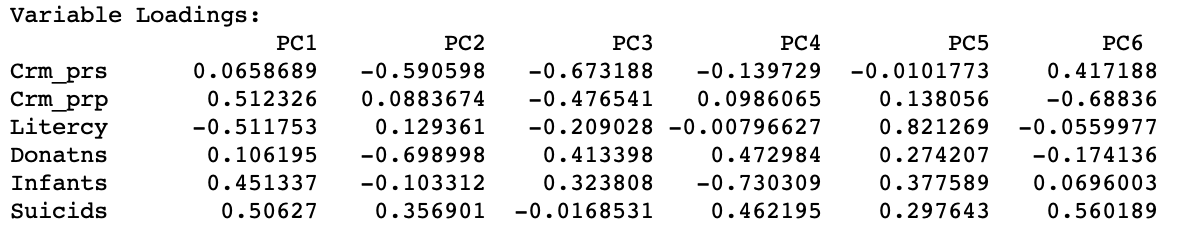For PC1, PC4, and PC6, the signs for the loadings are the opposite of those reported in Figure 10. This needs to be kept in mind when interpreting the actual value (and sign) of the components.

When the original variables are all standardized, each eigenvector coefficient gives a measure of the relative contribution of a variable to the component in question. These loadings are also the vectors employed in a principal component biplot, a common graph produced in a principal component analysis (but not currently available in GeoDa).

To further illustrate how the principal components are obtained, consider the standardized values for the six variables, listed in Figure 15 for the first five observations.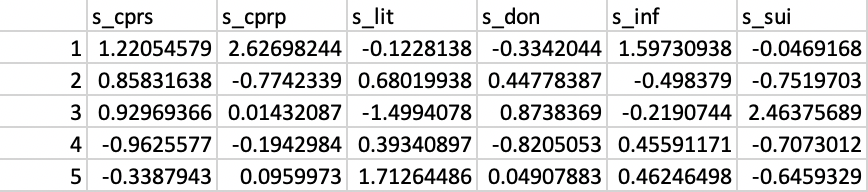Figure 15: Standardized variables

Each principal component is obtained by multiplying the values in the columns for each observation by the corresponding element of the eigenvector, listed as Variable Loadings in Figure 10 (using the results from the default option SVD). For the first principal component, the eigenvector is listed under PC1: -0.0659, -0.5123, 0.5118, -0.1062, -0.4513, -0.5063. For example, the value of the first principal component for the first observation is found as the product of the first row in Figure 15 with this eigenvector. Specifically, the result is 1.2205 $$\times$$ -0.0659 + 2.6270 $$\times$$ -0.5123 - 0.1228 $$\times$$ 0.5118 - 0.3342 $$\times$$ -0.1062 + 1.5973 $$\times$$ -0.4513 - 0.0469 $$\times$$ -0.5063 = - 2.1508, the value in the first row/column in Figure 13.

Similar calculations can be carried out to verify the other values.

#### Substantive interpretation of principal components

The interpretation and substantive meaning of the principal components can be a challenge. In factor analysis, a number of rotations are applied to clarify the contribution of each variable to the different components. The latter are then imbued with meaning such as “social deprivation”, “religious climate”, etc. Principal component analysis tends to stay away from this, but nevertheless, it is useful to consider the relative contribution of each variable to the respective components.

The table labeled as Squared correlations lists those statistics between each of the original variables in a row and the principal component listed in the column. Each row of the table shows how much of the variance in the original variable is explained by each of the components. As a result, the values in each row sum to one.

More insightful is the analysis of each column, which indicates which variables are important in the computation of the matching component. In our example, we see that Crm_prp, Litercy, Infants and Suicids are the most important for the first component, whereas for the second component this is Crm_prs and Donatns. As we can see from the cumulative proportion listing, these two components explain about 56% of the variance in the original variables.

Since the correlations are squared, they do not depend on the sign of the eigenvector elements, unlike the loadings.

#### Visualizing principal components

Once the principal components are added to the data table, they are available for use in any graph (or map).

A useful graph is a scatter plot of any pair of principal components. For example, such a graph is shown for the first two components (based on the SVD method) in Figure 16. By construction, the principal component variables are uncorrelated, which yields the characteristic circular cloud plot. A regression line fit to this scatter plot yields a horizontal line (with slope zero). Through linking and brushing, we can identify the locations on the map for any point in the scatter plot.

In addition, this type of bivariate plot is sometimes employed to visually identify clusters in the data. Such clusters would be suggested by distinct high density clumps of points in the graph. Such points are close in a multivariate sense, since they correspond to a linear combination of the original variables that maximizes explained variance.6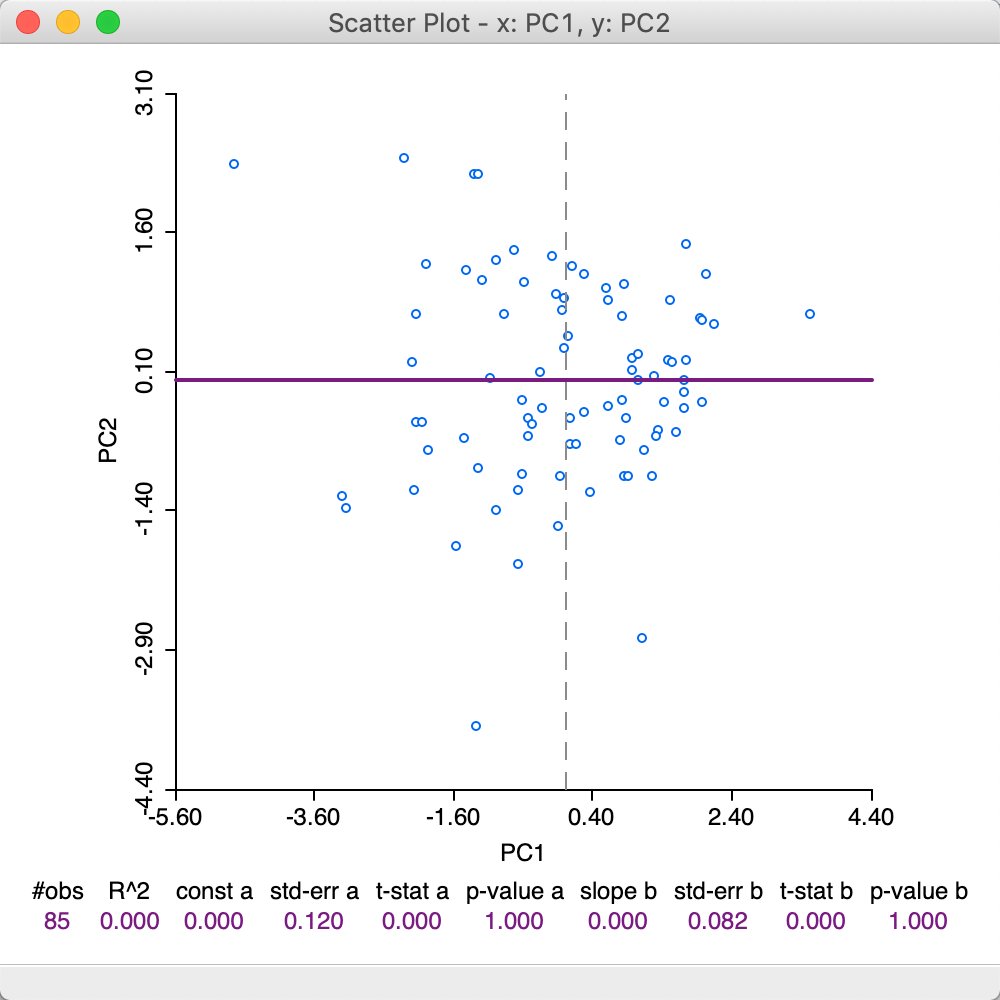Figure 16: First two principal components scatter plot - SVD method

To illustrate the effect of the choice of eigenvalue computation, the scatter plot in Figure 17 again plots the values for the first and second component, but now using the loadings from the Eigen method. Note how the scatter has been flipped around the vertical axis, since what used to be positive for PC1, is now negative, and vice versa.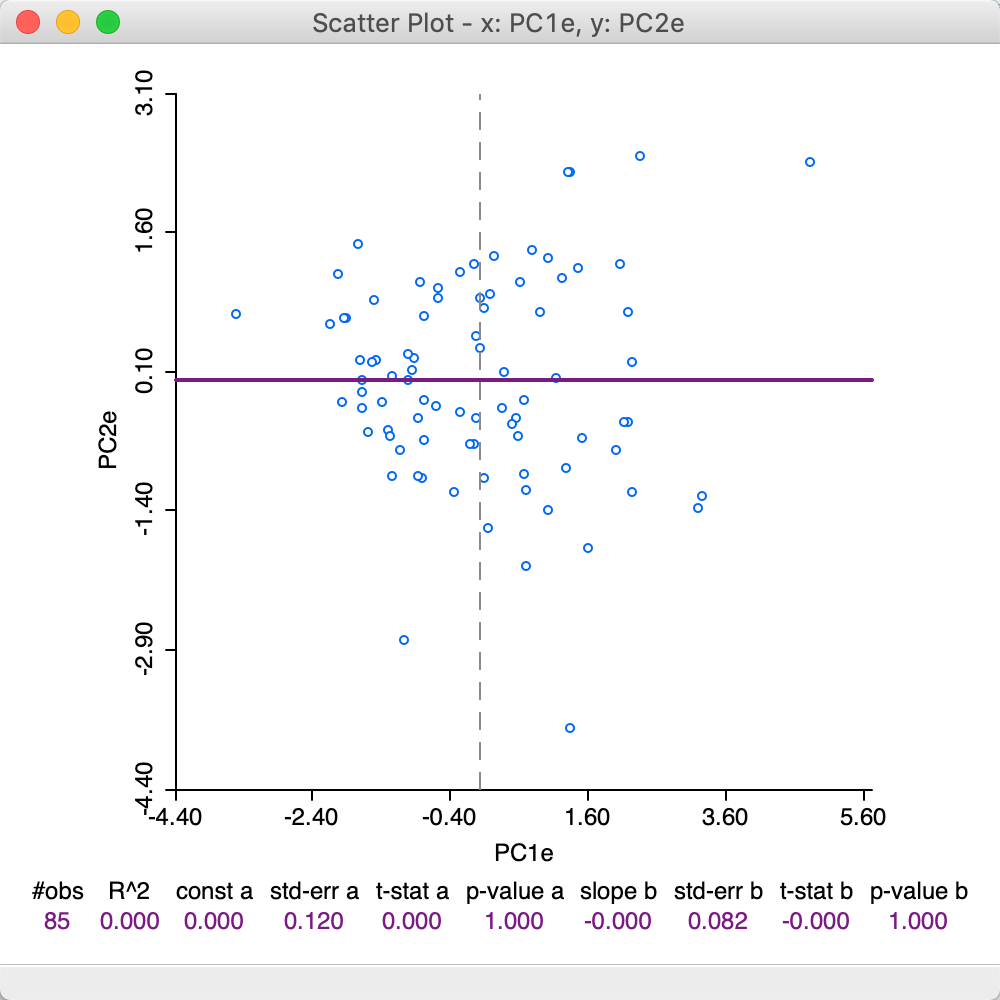Figure 17: First two principal components scatter plot - Eigen method

An even more graphic illustration of the difference between the principal components obtained through the SVD method (PC1) and the Eigen method (PC1e) can be seen by the perfect negative correlation in the scatter plot in Figure 16.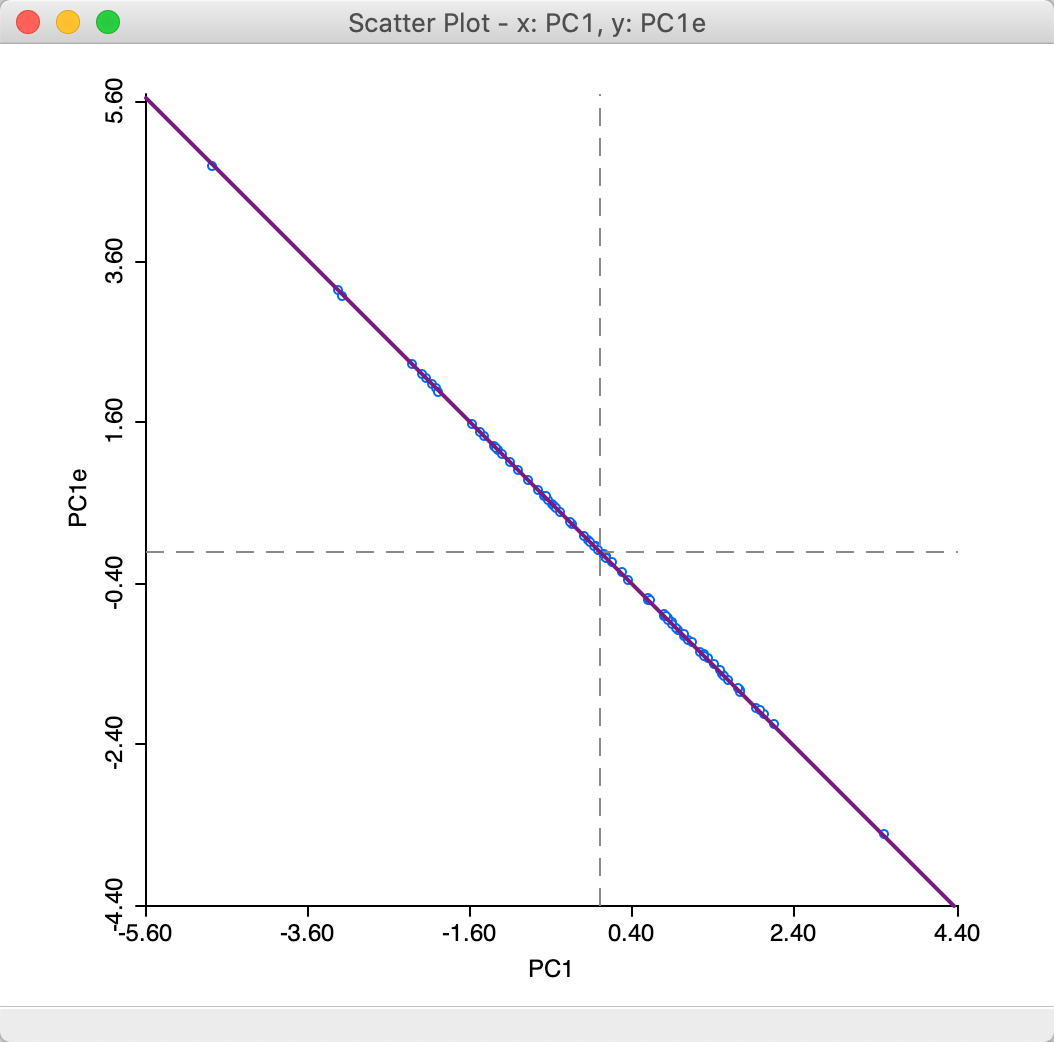Figure 18: First principal component for SVD and Eigen

In order to gain further insight into the composition of a principal component, we combine a box plot of the values for the component with a parallel coordinate plot of its main contributing variables. For example, in the box plot in Figure 19, we select the observations in the top quartile of PC1 (using SVD).7

We link the box plot to a parallel coordinate plot for the four variables that contribute most to this component. From the analysis above, we know that these are Crm_prp, Litercy, Infants and Suicids. The corresponding linked selection in the PCP shows low values for all but Litercy, but the lines in the plot are all close together. This nicely illustrates how the first component captures a clustering in multivariate attribute space among these four variables.

Since the variables are used in standardized form, low values will tend to have a negative sign, and high values a positive sign. The loadings for PC1 are negative for Crm_prp, Infants and Suicids, which, combined with negative standardized values, will make a (large) positive contribution to the component. The loadings for Litercy are positive and they multiply a (large) postive value, also making a positive contribution. As a result, the values for the principal component end up in the top quartile.Figure 19: PC1 composition using PCP

The substantive interpretation is a bit of a challenge, since it suggests that high property crime, out of wedlock births and suicide rates (recall that low values for the variables are bad, so actually high rates) coincide with high literacy rates (although the latter are limited to military conscripts, so they may be a biased reflection of the whole population).

## Spatializing Principal Components

We can further spatialize the visualization of principal components by explicitly considering their spatial distribution in a map. In addition, we can explore spatial patterns more formally through a local spatial autocorrelation analysis.

### Principal component maps

The visualization of the spatial distribution of the value of a principal component by means of a choropleth map is mostly useful to suggest certain patterns. Caution needs to be used to interpret these in terms of high or low, since the latter depends on the sign of the component loadings (and thus on the method used to compute the eigenvectors).

For example, using the results from SVD for the first principal component, a quartile map shows a distinct pattern, with higher values above a diagonal line going from the north west to the middle of the south east border, as shown in Figure 20.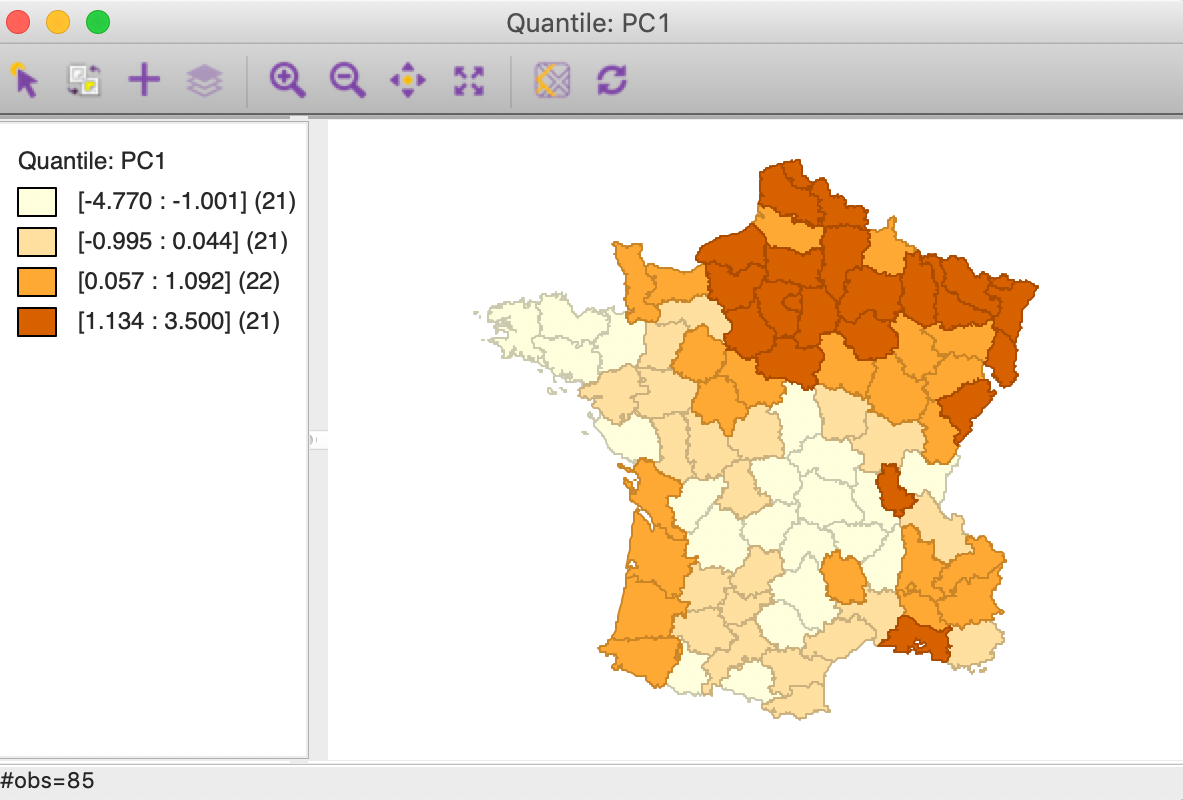Figure 20: PC1 quartile map

A quartile map for the first component computed using the Eigen method shows the same overall spatial pattern, but the roles of high and low are reversed, as in Figure 21.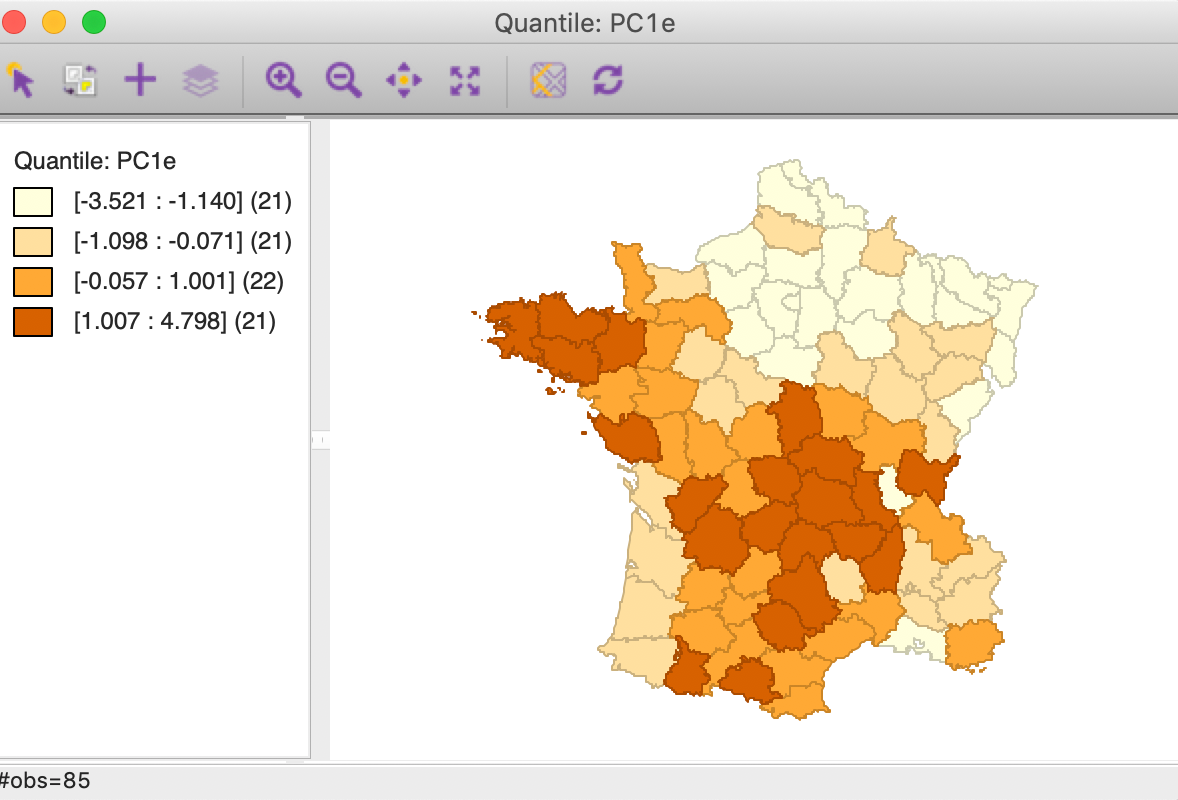Figure 21: PC1 quartile map

Even clearer evidence of the need for care in the interpretation of the components is seen in Figures 22 and 23 that show a box map for the first principal component, respectively for SVD and for Eigen. What is shown as a lower outlier in for SVD is an upper outlier in the box map for Eigen.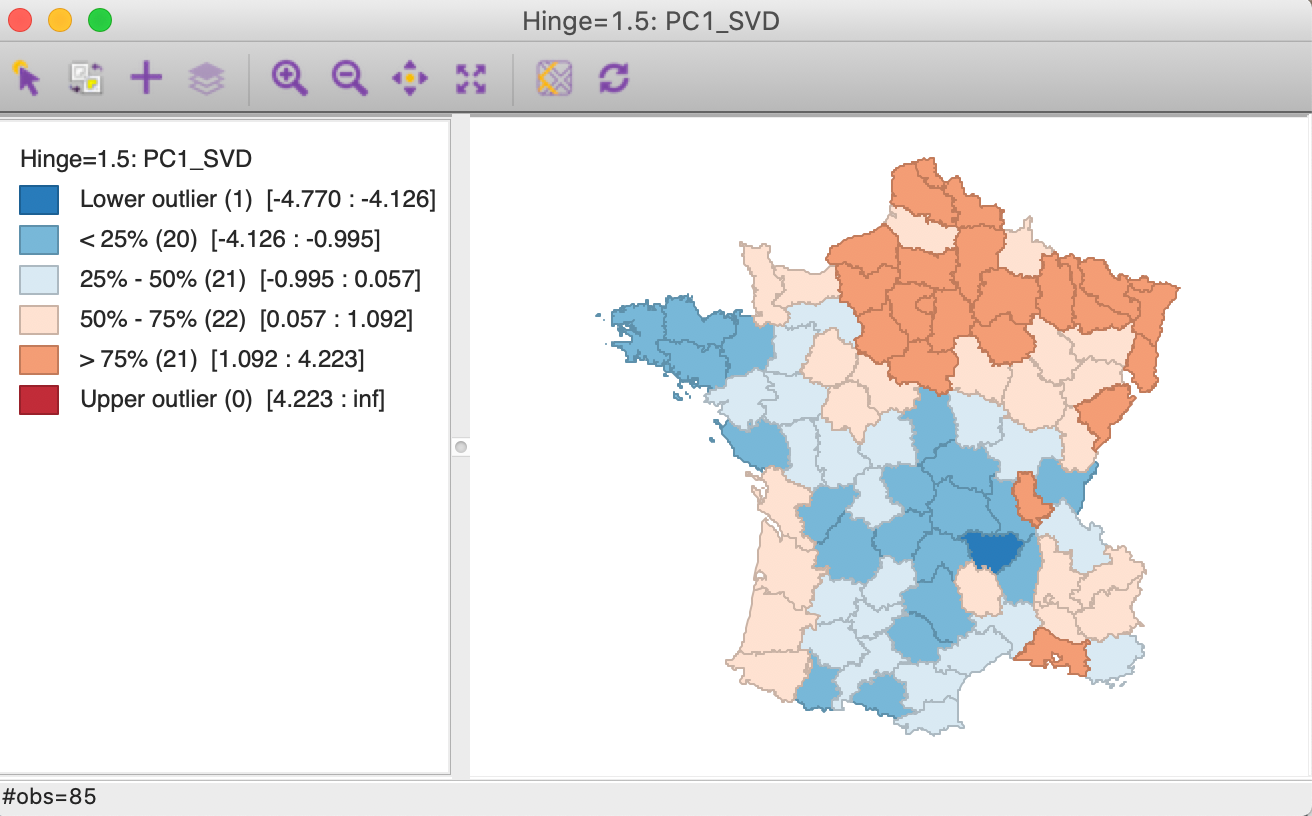Figure 22: PC1-SVD box map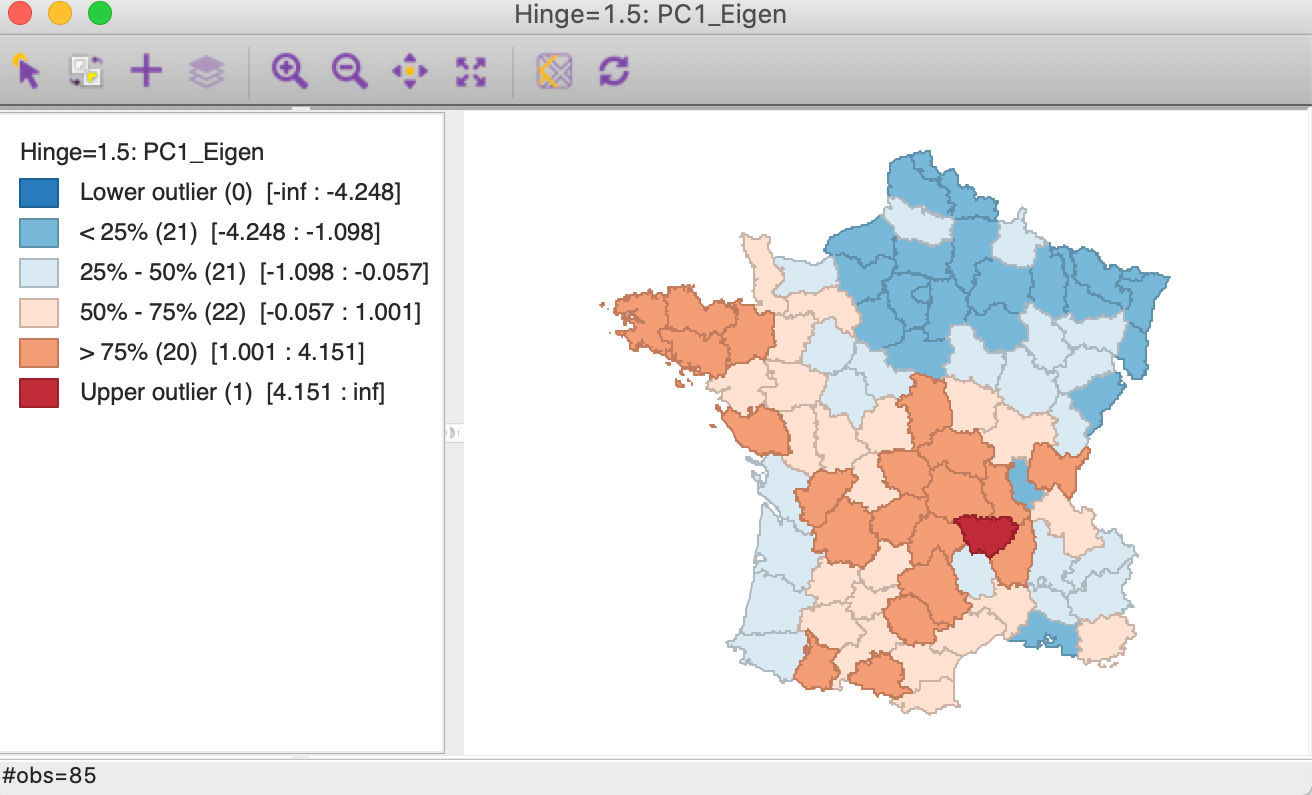Figure 23: PC1-Eigen box map

### Cluster maps

We pursue the nature of this pattern more formally through a local Moran cluster map. Again, using the first component from the SVD method, we find a strong high-high cluster in the northern part of the country, ranging from east to west (with p=0.01 and 99999 permutations, using queen contiguity). In addition, there is a pronounced Low-Low cluster in the center of the country. With the component based on the Eigen method, the locations of the clusters would be the same, but their labels would be opposite, i.e., what was high-high becomes low-low and vice versa. In other words, the location of the clusters is not affected by the method used, but their interpretation is.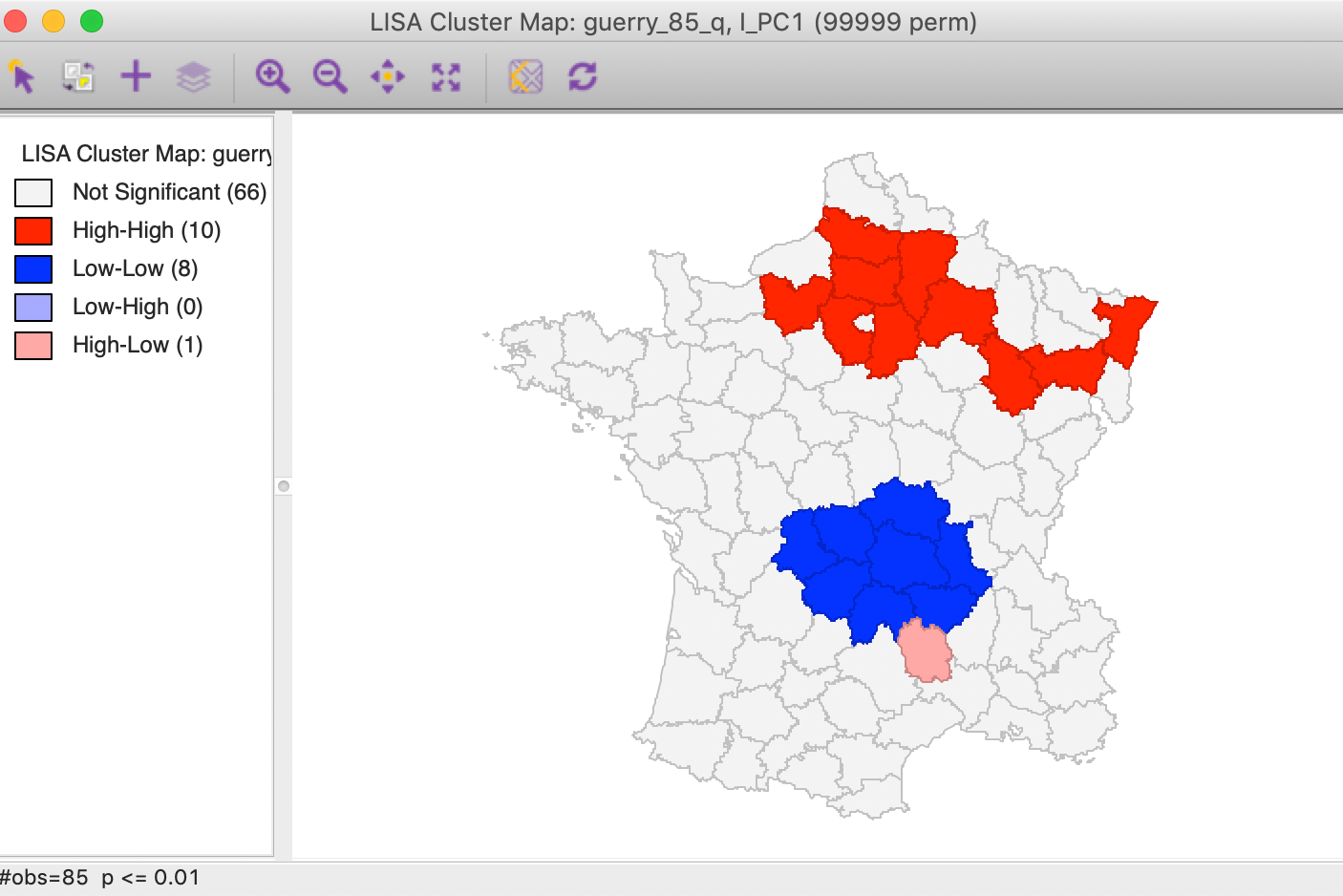Figure 24: PC1 Local Moran cluster map

### Principal components as multivariate cluster maps

Since the principal components combine the original variables, their patterns of spatial clustering could be viewed as an alternative to a full multivariate spatial clustering. The main difference is that in the latter each variable is given equal weight, whereas in the principal component some variables are more important than others. In our example, we saw that the first component is a reasonable summary of the pattern among four variables, Crm_prp, Litercy, Infants and Suicids.

First, we can check on the makeup of the component in the identified cluster. For example, we select the high-high values in the cluster map and find the matching lines in a parallel coordinate plot for the four variables, shown in Figure 25. The lines track closely (but not perfectly), suggesting some clustering of the data points in the four-dimensional attribute space. In other words, the high-high spatial cluster of the first principal component seems to match attribute similarity among the four variables.Figure 25: Linked PCA cluster map and PCP

In addition, we can now compare these results to a cluster or significance map from a multivariate local Geary analysis for the four variables. In Figure 26, we show the significance map rather than a cluster map, since all significant locations are for positive spatial autocorrelation (p < 0.01, 99999 permutations, queen contiguity). While the patterns are not identical to those in the principal component cluster map, they are quite similar and pick up the same general spatial trends.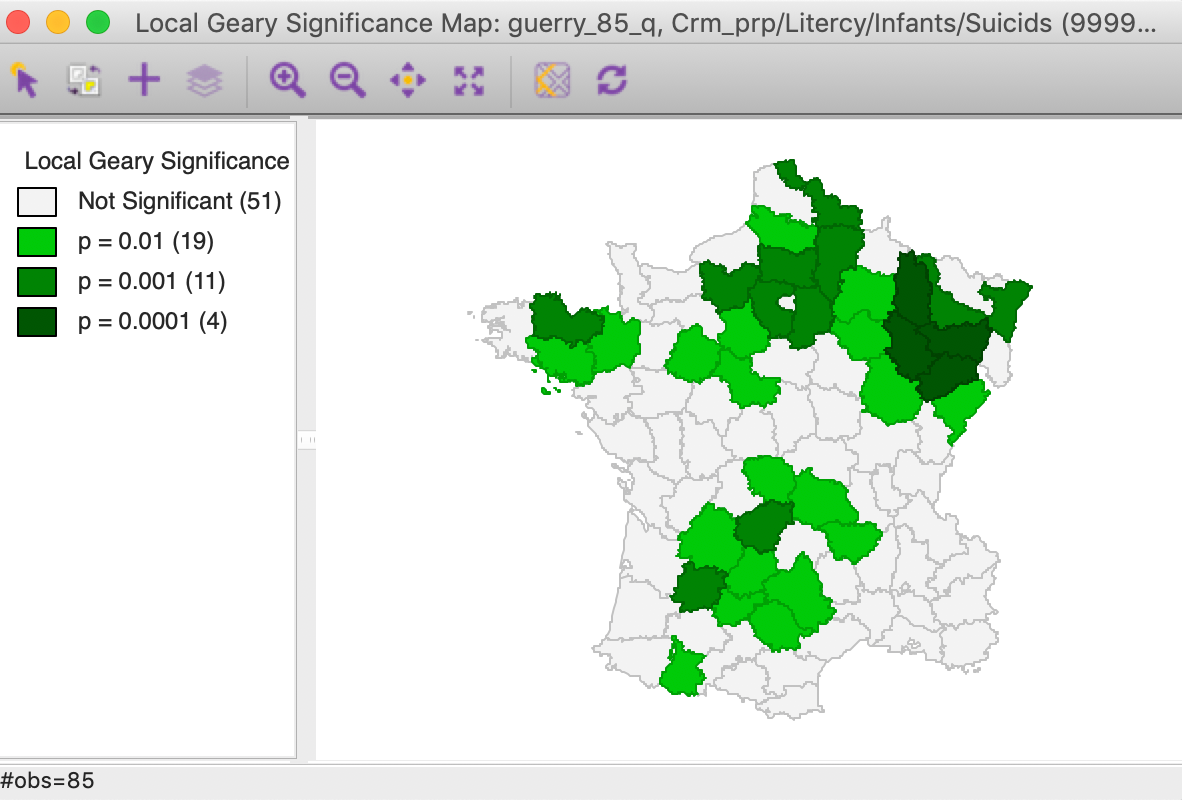Figure 26: Multivariate local Geary significance map

This suggests that in some instances, a univariate local spatial autocorrelation analysis for one or a few dominant principal components may provide a viable alternative to a full-fledged multivariate analysis.

## Appendix

### Basics - eigenvalues and eigenvectors

Consider a square symmetric matrix:8 $A = \begin{bmatrix} 1 & 3\\ 3 & 2 \end{bmatrix}$ and a column vector, say: $v = \begin{bmatrix} 1\\ 2 \end{bmatrix}$ As illustrated in Figure 27, we can visualize the vector as an arrow from the origin of a $$x-y$$ scatter plot going to the point ($$x = 1$$, $$y = 2$$).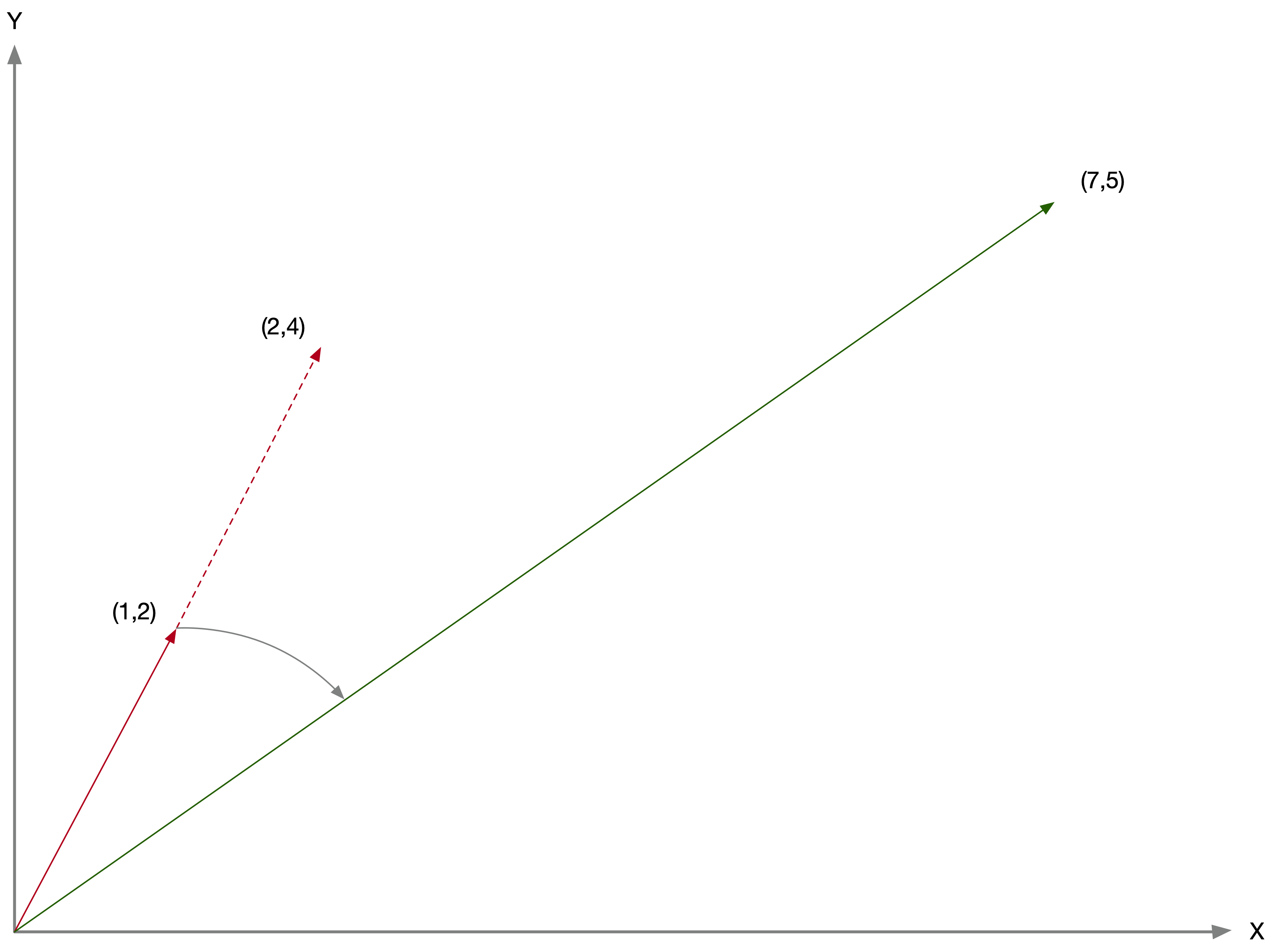Figure 27: Vectors in two-dimensional space

Pre-multiplying this vector by a scalar just moves the point further on the same slope. For example, $2 \times \begin{bmatrix} 1\\ 2 \end{bmatrix} = \begin{bmatrix} 2\\ 4 \end{bmatrix}$ This is equivalent to moving the arrow over on the same slope from (1,2) to the point (2,4) further from the origin, as shown by the dashed line in Figure 27.

In contrast, pre-multiplying the vector by the matrix does both rescaling and rotation of the vector. In our example, $Av = \begin{bmatrix} (1 \times 1) + (3 \times 2)\\ (3 \times 1) + (2 \times 2) \end{bmatrix} = \begin{bmatrix} 7 \\ 5 \end{bmatrix}.$ In Figure 27, the arrow is both moved further away from the origin and also rotated down to the point (7,5) (now the x-value is larger than the y-value, so the slope of the arrow is less steep).

The eigenvectors and eigenvalues of a square symmetric matrix are a special pair of scalar-vector, such that $$Av = \lambda v$$, where $$\lambda$$ is the eigenvalue and $$v$$ is the eigenvector. In addition, the eigenvectors are orthogonal to each other, i.e., $$v_u'v_k = 0$$ (for different eigenvectors), and $$v_u'v_u = 1$$ (the sum of the squares of the eigenvector elements sum to one).

What does this mean? For this particular vector (i.e., arrow from the origin), the transformation by $$A$$ does not rotate the vector, but simply rescales it (i.e., moves it further or closer to the origin), by exactly the factor $$\lambda$$. In our example, the two eigenvectors turn out to be [0.6464 0.7630] and [-0.7630 0.6464], with associated eigenvalues 4.541 and -1.541. Each square matrix has as many eigenvectors and matching eigenvalues as its rank, in this case 2 – for a 2 by 2 nonsingular matrix. The actual computation of eigenvalues and eigenvectors is rather complicated, so let’s just assume we have the result.

Consider post-multiplying the matrix $$A$$ with the eigenvector [0.6464 0.7630]: $\begin{bmatrix} (1 \times 0.6464) + (3 \times 0.7630)\\ (3 \times 0.6464) + (2 \times 0.7630) \end{bmatrix} = \begin{bmatrix} 2.935\\ 3.465 \end{bmatrix}$ Now, for comparison purposes, we rescale the eigenvector with the matching eigenvalue: $4.541 \times \begin{bmatrix} 0.6464\\ 0.7630 \end{bmatrix} = \begin{bmatrix} 2.935\\ 3.465 \end{bmatrix}$ This yields the same result as the matrix multiplication.

Furthermore, if we stack the eigenvectors in a matrix $$V$$, we can verify that they are orthogonal and the sum of squares of the coefficients sum to one, i.e., $$V'V = I$$: $\begin{bmatrix} 0.6464 & -0.7630\\ 0.7630 & 0.6464 \end{bmatrix}' \begin{bmatrix} 0.6464 & -0.7630\\ 0.7630 & 0.6464 \end{bmatrix} = \begin{bmatrix} 1 & 0\\ 0 & 1 \end{bmatrix}$ In addition, it is easily verified that $$VV' = I$$ as well. This means that the transpose of $$V$$ is also its inverse (per the definition of an inverse matrix, i.e., a matrix for which the product with the original matrix yields the identity matrix), or $$V^{-1} = V'$$.

As a result, we have found a particular vector (and associated scalar) so that multiplying this vector by $$A$$ does not result in any rotation, but only in a rescaling.

Eigenvectors and eigenvalues are central in many statistical analyses, but it is important to realize they are not as complicated as they may seem at first sight. On the other hand, computing them efficiently is complicated, and best left to specialized programs (GeoDa uses the Eigen C++ library for these computations, http://eigen.tuxfamily.org/).

Finally, a couple of useful properties of eigenvalues are worth mentioning.

The sum of the eigenvalues equals the trace of the matrix. The trace is the sum of the diagonal elements, in our example, the trace is $$1 + 2 = 3$$. The sum of the two eigenvalues is $$4.541 -1.541 = 3$$. Also, the product of the eigenvalues equals the determinant of the matrix. For a $$2 \times 2$$ matrix, the determinant is $$ab - cd$$, or the product of the diagonal elements minus the product of the off-diagonal elements. In our example, that is $$(1 \times 2) - (3 \times 3) = -7$$. The product of the two eigenvalues is $$4.541 \times -1.541 = -7.0$$.

### Eigenvector decomposition of a square matrix

For each eigenvector $$v$$ of a square symmetric matrix $$A$$, we have $$Av = \lambda v$$. We can write this compactly for all the eigenvectors and eigenvalues by organizing the individual eigenvectors as columns in a $$k \times k$$ matrix $$V$$, as we have seen above. Similarly, we can organize the matching eigenvalues as the diagonal elements of a diagonal matrix, say $$G$$. The basic eigenvalue expression can then be written as $$AV = VG$$. Note that $$V$$ goes first in the matrix multiplication on the right hand side to ensure that each column of $$V$$ is multiplied by the corresponding eigenvalue on the diagonal of $$G$$ to yield $$\lambda v$$. Now, we take advantage of the fact that the eigenvectors are orthogonal, namely that $$VV' = I$$. Post-multiplying each side of the equation by $$V'$$ gives $$AVV' = VGV'$$, or $$A = VGV'$$, the so-called eigenvalue decomposition or spectral decomposition of a square symmetric matrix.

Since the correlation matrix $$X'X$$ is square and symmetric, its spectral decomposition has the same structure: $X'X = VGV',$ with $$V$$ and $$G$$ have the same meaning as before. In the context of principal component analysis, the coefficients $$a_h$$ needed to construct the component from the original variables are the elements of the eigenvector column, with the row matching the column of the original variable. For example, the coefficients $$a_2$$ needed to multiply the variable $$x_2$$ would be in the second row of the matrix $$V$$.

The variance associated with the h-th principal component is the h-th element on the diagonal of $$G$$. With these values in hand, we have all the information to construct and interpret the principal components.

In GeoDa, these computations are carried out by means of the EigenSolver routine from the Eigen C++ library (https://eigen.tuxfamily.org/dox/classEigen_1_1EigenSolver.html).

### Singular Value Decomposition (SVD)

The eigenvalue decomposition described above only applies to square matrices. A more general decomposition is the so-called singular value decomposition, which applies to any rectangular matrix, such as the $$n \times k$$ matrix $$X$$ with (standardized) observations.

For a full-rank $$n \times k$$ matrix $$X$$ (there are many more general cases where SVD can be applied), the decomposition takes the following form: $X = UDV',$ where $$U$$ is a $$n \times k$$ orthonormal matrix (i.e., $$U'U = I$$), $$D$$ is a $$k \times k$$ diagonal matrix, and $$V$$ is a $$k \times k$$ orthonormal matrix (i.e., $$V'V = I$$). In essence, the decomposition shows how a transformation $$X$$ applied to a vector $$y$$ (through a matrix multiplication of $$X$$ with a $$k$$-dimensional vector $$y$$) can be viewed to consist of a series of rotations and rescalings. Note that in the general rectangular case, the transformation $$Xy$$ not only involves rotation and rescaling (as in the square case), but also a change in dimensionality from a $$k$$-dimensional space (the length of $$y$$) to an $$n$$-dimensional space (the length of $$Xy$$, i.e., an n-dimensional vector). An intuitive visualization of the operations involved in such a transformation that are reflected in the elements of the SVD can be found at https://en.wikipedia.org/wiki/File:Singular_value_decomposition.gif.

While SVD is very general, we don’t need that full generality for the PCA case. It turns out that there is a direct connection between the eigenvalues and eigenvectors of the (square) matrix $$X'X$$ and the SVD of $$X$$. Using the expression above, we can write $$X'X$$ as: $X'X = (UDV')'(UDV') = VDU'UDV' = VD^2V',$ since $$U'U = I$$.

It thus turns out that the columns of $$V$$ from the SVD contain the eigenvectors of $$X'X$$ and the square of the diagonal elements of $$D$$ in the SVD are the eigenvalues associated with the corresponding principal component.

The concept of singular value decomposition is very broad and a detailed discussion is beyond our scope. A number of computational approaches are available. In GeoDa, the JacobiSVD routine is used from the Eigen C++ library (https://eigen.tuxfamily.org/dox/classEigen_1_1JacobiSVD.html). Note that other software (such as R’s pca) uses an algorithm based on the Householder transformation (from the lapack library). This yields the opposite signs for the eigenvector elements.

1. University of Chicago, Center for Spatial Data Science – ↩︎

2. In GeoDa, this can be accomplished in the weights manager, using the variables option for distance weights, and making sure the transformation is set to raw. The nearest neighbor distances are the third column in a weights file created for $$k = 1$$.↩︎

3. The standardization should not be done mechanically, since there are instances where the variance differences between the variables are actually meaningful, e.g., when the scales on which they are measured have a strong substantive meaning (e.g., in psychology).↩︎

4. Since the eigenvalues equal the variance explained by the corresponding component, the diagonal elements of $$D$$ are thus the standard deviation explained by the component.↩︎

5. The concept of reconstruction error is somewhat technical. If $$A$$ were a square matrix, we could solve for $$z$$ as $$z = A^{-1}x$$, where $$A^{-1}$$ is the inverse of the matrix $$A$$. However, due to the dimension reduction, $$A$$ is not square, so we have to use something called a pseudo-inverse or Moore-Penrose inverse. This is the $$p \times k$$ matrix $$(A'A)^{-1}A'$$, such that $$z = (A'A)^{-1}A'x$$. Furthermore, because $$A'A = I$$, this simplifies to $$z = A'x$$ (of course, so far the elements of $$A$$ are unknown). Since $$x = Az$$, if we knew what $$A$$ was, we could find $$x$$ as $$Az$$, or, with our solution in hand, as $$AA'x$$. The reconstruction error is then the squared difference between $$x$$ and $$AA'x$$. We have to find the coeffients for $$A$$ that minimize this expression. For an extensive technical discussion, see Lee and Verleysen (2007), Chapter 2.↩︎

6. For an example, see, e.g., Chapter 2 of Everitt et al. (2011).↩︎

7. Note that, when using the Eigen method, these would be the bottom quartile of the first component.↩︎

8. Not all the results for symmetric matrices hold for asymmetric matrices. However, since we only deal with symmetric matrices in the applications covered here, we just stick to the simpler case.↩︎